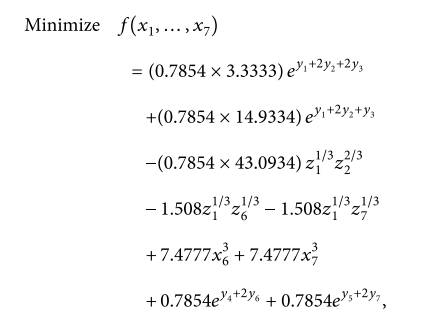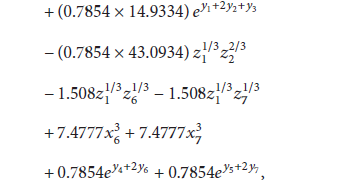# Weight minimization of a speed reducer

or just write the code.Also give me some link for cvx tutorials.
The objective of Golinski’s Speed Reducer problem is to find the minimum of a gear box volume f (and, hence, its minimum weight), subject to several constraints. There are seven design variables, x1 - x7 , which represent

x1 width of the gear face, cm
x2 teeth module, cm
x3 number of pinion teeth
x4 shaft 1 length between bearings, cm
x5 shaft 2 length between bearings, cm
x6 diameter of shaft 1, cm
x7 diameter of shaft 2, cm

Mathematically, the problem is specified as follows:I doubt this is a convex problem. For instance g_5 does not look convex because that would have negative value in the Hessian for x_6. Right?

Except for some unfortunate terms, as @Erling pointed out, this would be a Geometric Program which CVX could handle. See http://cvxr.com/cvx/doc/gp.html and read https://web.stanford.edu/~boyd/papers/gp_tutorial.html for a more in depth understanding.

But his isn’t horseshoes, hand grenades, or nuclear weapons, so close doesn’t count.

The CVX Users’ Guide is the tutorial http://cvxr.com/cvx/doc/ . The are CVX examples at http://cvxr.com/cvx/examples/ as well as in this forum.

so is this non-convex?

You should prove to us that the problem is convex. That is your job but we think it is not convex.

i have re-written the problem by transforming it into convex MINLP. now this is convex and a geomtric program. kindly help with the codeHow did you transform this into a convex MINLP?